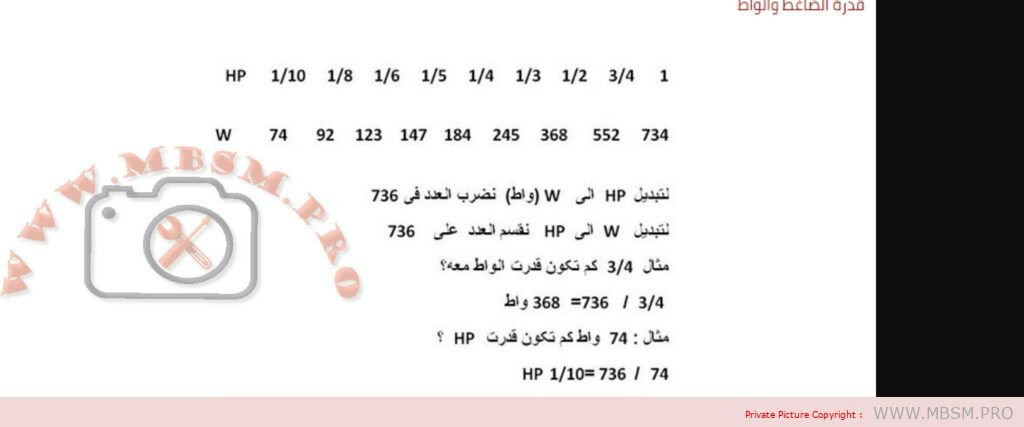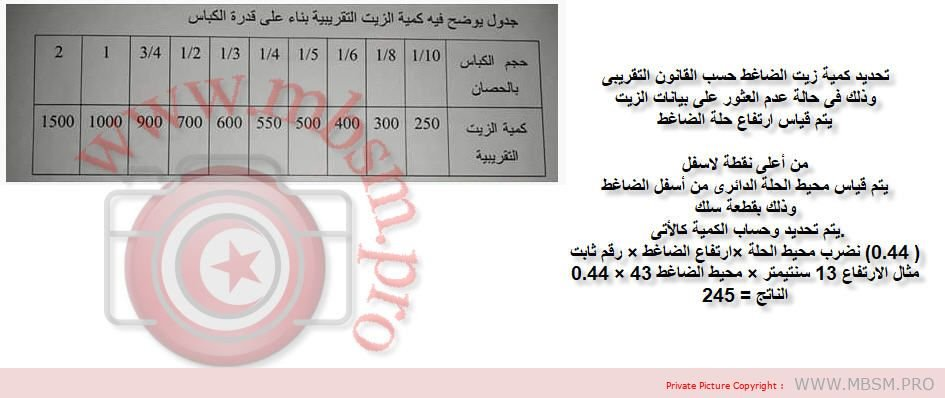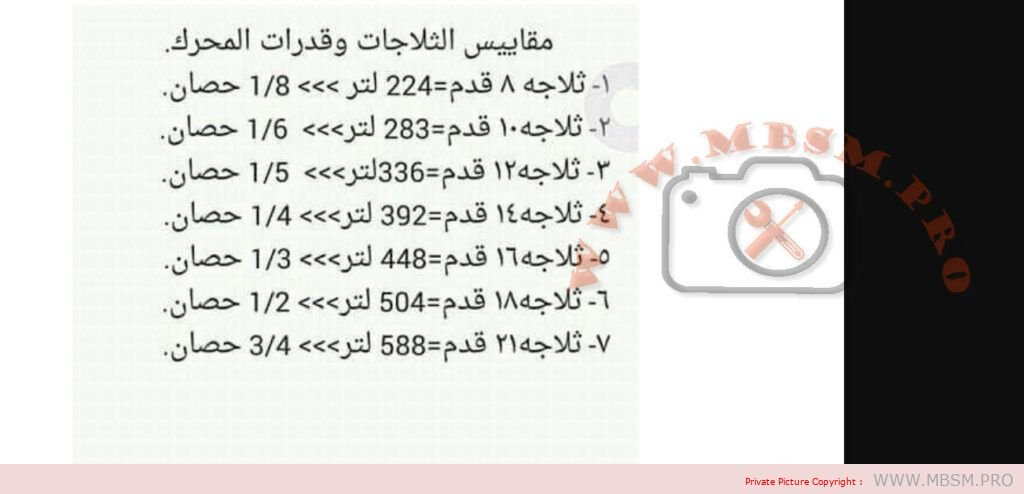Convert - MBSM DOT PRO
Welcome to Mbsm.pro, Magistral, Best, Secure, More and professional informations...

# Convert

• 1 Kcal/hr = 1.163 W
• 1 Hp = 746 W
• 1 Ton = 12000 BTU = 3.517 Wk = 3517 W = 3040 KCAL/H
• 1 Hp = 8000 BTU
• 26000 BTU = 2 Ton
• 16000 BTU = 1 1/2 Ton
• KCal/h * 1.163 = Watt
•  KCal/h * 3.968 = Btu/h
•  W * 3.412 = Btu/h
•  W * 0.86 = Kcal/H

## HVAC metrics:

SEER = (1 × EER100% + 42 × EER75% + 45 × EER50% + 12 × EER250%)/100

EER = BTUcooling/W

HSPF = BTUheating/W

COP = Q (useful heat)/W (input work)

ACH = CFM x 60/Area x Heightceiling

Total Heat (BTU/hr.) = 4.5 x CFM x Δh (std. air)

Sensible Heat (BTU/hr) = 1.1 x CFM x Δt (std. air)

Latent Heat (BTU/hr) = 0.69 x CFM x Δgr. (std. air)

1 HP = 746 Watts

1 kW = 3413 BTU